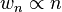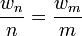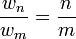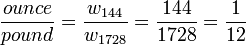## Weight and amount

Weight, by definition, is a measure of the force which must be applied to support an object (i.e. hold it at rest) in a gravitational field. The Earth’s gravitational field causes items near the Earth to have weight. Typically, gravitational fields change only slightly over short distances, and the Earth’s field is nearly uniform at all locations on the Earth’s surface; therefore, an object’s weight changes only slightly when it is moved from one location to another, and these small changes went unnoticed through much of history. This may have given early humans the impression that weight is an unchanging, fundamental property of objects in the material world.

In the Egyptian religious illustration to the above, Anubis is using a balance scale to weigh the heart of Hunefer. A balance scale balances the force of one object’s weight against the force of another object’s weight. The two sides of a balance scale are close enough that the objects experience similar gravitational fields. Hence, if they have similar masses then their weights will also be similar. The scale, by comparing weights, also compares masses. The balance scale is one of the oldest known devices for measuring mass.

The concept of amount is very old and predates recorded history, so any description of the early development of this concept is speculative in nature. However, one might reasonably assume[citation needed] that humans, at some early era, realized that the weight of a collection of similar objects was directly proportional to the number of objects in the collection:$w_n \propto n$,
where w is the weight of the collection of similar objects and n is the number of objects in the collection. Proportionality, by definition, implies that two values have a constant ratio:$\frac{w_n}{n} = \frac{w_m}{m}$, or equivalently$\frac{w_n}{w_m} = \frac{n}{m}$.
Consequently, historical weight standards were often defined in terms of amounts. The Romans, for example, used the carob seed (carat or siliqua) as a measurement standard. If an object’s weight was equivalent to 1728 carob seeds, then the object was said to weigh one Roman pound. If, on the other hand, the object’s weight was equivalent to 144 carob seeds then the object was said to weigh one Roman ounce (uncia). The Roman pound and ounce were both defined in terms of different sized collections of the same common mass standard, the carob seed. The ratio of a Roman ounce (144 carob seeds) to a Roman pound (1728 carob seeds) was:$\frac{ounce}{pound} = \frac{w_{144}}{w_{1728}} = \frac{144}{1728} = \frac{1}{12}$.
This example illustrates a fundamental principle of physical science: when values are related through simple fractions, there is a good possibility that the values stem from a common source.

The name atom comes from the Greek ἄτομος/átomos, α-τεμνω, which means uncuttable, something that cannot be divided further. The philosophical concept that matter might be composed of discrete units that cannot be further divided has been around for millennia. However, empirical proof and the universal acceptance of the existence of atoms didn’t occur until the early 20th century.

As the science of chemistry matured, experimental evidence for the existence of atoms came from the law of multiple proportions. When two or more elements combined to form a compound, their masses are always in a fixed and definite ratio. For example, the mass ratio of nitrogen to oxygen in nitric oxide is seven eights. Ammonia has a hydrogen to nitrogen mass ratio of three fourteenths. The fact that elemental masses combined in simple fractions implies that all elemental mass stems from a common source. In principle, the atomic mass situation is analogous to the above example of Roman mass units. The Roman pound and ounce were both defined in terms of different sized collections of carob seeds, and consequently, the two mass units were related to each other through a simple fraction. Comparatively, since all of the atomic masses are related to each other through simple fractions, then perhaps the atomic masses are just different sized collections of some common fundamental mass unit.

In 1805, the chemist John Dalton published his first table of relative atomic weights, listing six elements, hydrogen, oxygen, nitrogen, carbon, sulfur, and phosphorus, and assigning hydrogen an atomic weight of 1. And in 1815, the chemist William Prout concluded that the hydrogen atom was in fact the fundamental mass unit from which all other atomic masses were derived.

If Prout's hypothesis had proven accurate, then the abstract concept of mass, as we now know it, might never have evolved, since mass could always be defined in terms of amounts of the hydrogen atomic mass. Prout’s hypothesis; however, was found to be inaccurate in two major respects. First, further scientific advancements revealed the existence of smaller particles, such as electrons and quarks, whose masses are not related through simple fractions. And second, the elemental masses themselves were found to not be exact multiples of the hydrogen atom mass, but rather, they were near multiples. Einstein’s theory of relativity explained that when protons and neutrons come together to form an atomic nucleus, some of the mass of the nucleus is released in the form of binding energy. The more tightly bound the nucleus, the more energy is lost during formation and this binding energy loss causes the elemental masses to not be related through simple fractions.
Hydrogen, for example, with a single proton, has an atomic weight of 1.007825 u. The most abundant isotope of iron has 26 protons and 30 neutrons, so one might expect its atomic weight to be 56 times that of the hydrogen atom, but in fact, its atomic weight is only 55.9383 u, which is clearly not an integer multiple of 1.007825. Prout’s hypothesis was proven inaccurate in many respects, but the abstract concepts of atomic mass and amount continue to play an influential role in chemistry, and the atomic mass unit continues to be the unit of choice for very small mass measurements.

When the French invented the metric system in the late 18th century, they used an amount to define their mass unit. The kilogram was originally defined to be equal in mass to the amount of pure water contained in a one-liter container. This definition, however, was inadequate for the precision requirements of modern technology, and the metric kilogram was redefined in terms of a manmade platinum-iridium bar known as the international prototype kilogram.